# Log-normal diameter distribution of Pd-based metallic glass droplet and wire

## Abstract

We have studied the formation of Pd42.5Cu30Ni7.5P20 metallic glass droplets and wires in the gas atomization process. We demonstrate that the sizes of droplets and wires can be distinguished by the Ohnesorge number (Oh), which is the proportion of the spinnability to the capillary instability and the diameter distributions follow a log-normal distribution function, implying cascade fragmentation. For droplets, the number significantly increases at Oh < 1 but the diameter gradually decreases. For wires, the number greatly increases at Oh > 1 while the diameter steadies below 400 nm. Further, the wire diameter is quadrupled at Oh = 16 due to the high viscosity which suppresses both capillary breakup and ligament elongation.

## Introduction

Nanostructures of metallic glasses (MGs) have attracted growing interests in materials science and technology. MG nanowires are promising building blocks for magnetic sensors, battery electrodes and heterogeneous catalysts1,2,3,4,5,6,7. The development for practical applications requires a large amount of the high quality MG wires with low cost. Recently, Nakayama et al. have succeeded in producing MG nanowires using the gas atomization process that has been a powerful method for producing metal and alloy powders. However, the fragmentation of molten alloys during the gas atomization processes is complicated because it occurs under the non-equilibrium states before solidification. Therefore, the fundamental understanding of fragmentation processes using fluid mechanics is important for controlling sizes and shapes. There have been extensive studies for gas atomization in powder metallurgy8. In general, the primary breakup in the atomization creates droplets at the molten alloy surface of melted streams. The secondary breakup produces subsequent droplets and further cascade fragmentation creates fine droplets, which leads to the broad size distribution10. However, much less attention has been paid to filamentary structures such as Pele’s (the Hawaiian goddess of volcanoes) hair11 though the difference is simply achieved by the spinnability, which is defined as an ability to form into threads or fibers12.

The breakup of viscous ligaments through the capillary instability within the time scale tcap can be described by13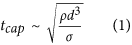where d is the ligament diameter, σ is the surface tension and ρ is the density. In contrast, the time scale of the spinnability, tvis attributed by the viscous forces is given by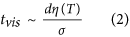where η is the viscosity. Consequently, the Ohnesorge number (Oh) is obtained by the ratio of these time scales as14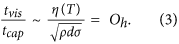Generally, when tvis/tcap = Oh < 1, the capillary instability dominates and the fragmentation into droplets becomes a favored process. In contrast, when Oh > 1, the spinnability dominates and the ligaments can be stretched before their solidification.

In this paper, we study the diameter distribution of droplets and wires composed of Pd42.5Cu30Ni7.5P20 metallic glass (Pd-MG). Pd-MG was used because it has a high glass forming ability and a high resistance to the oxidation15. Based on the Angell plot16, the viscosity of MGs have been described in the supercooled liquid region, which is defined by the difference between the crystallization temperature (Tx) and the grass transition temperature (Tg) and the temperature dependence in thermoplastic formability has been discussed, previously17,18. We focus on the viscosity near the melting point (Tm) where it significantly increases when the sample is supercooled from Tm. Therefore, the viscosity-dominated process associated with high Ohnesorge number plays an important role in the wire elongation. The aim of this study is fundamental understanding of the formation rule for the MG nanowires and microwires and would be tailoring length, diameter and distribution. The temperature is a key parameter because it associates with the viscosity. We have created droplets and wires with varying temperature and obtained the diameter distribution by counting them. We found that the distribution follows a log-normal distribution, indicating cascade fragmentation events in the gas atomization. The formation of thicker wires is dominated at Oh > 1 which can be realized only below Tm.

## Results

The Pd-MG sample was heated by an induction heating coil in the gas atomization system (Fig. 1). The induction heating temperature (Tin) is varied as a parameter in this study (See Methods). Figure 2 shows the results of energy dispersive X-ray spectroscopy (EDX), X-ray diffraction (XRD) and differential scanning calorimeter (DSC) for the wires created at Tin = 773 K. The EDX spectrum in Fig. 2a identify Pd, Cu, Ni, P, C and O elements, which are equivalent to the original composition, revealing that the incorporation of contaminations during the atomization process is negligible. The gas atomization produces a large amount of droplets and wires that allows conventional XRD and DSC measurements. The XRD pattern is obtained from about 10 mg sample containing mostly wires (cf. Fig. 3f), showing two broad halos corresponding to a fully amorphous phase, as shown in Fig. 2b. The DSC result obtained from about 3 mg of the sample indicates Tg = 570 K and Tx = 655 K, as shown in Fig. 2c. These results are consistent with those of bulk Pd-MG19 and the glassy phase of the atomized products is sustained. However, there is significant structural relaxation. The red curve in Fig. 2c corresponds to the first scan up to 570 K < Tg. After the first scan, we repeat the scans with four times below 570 K to induce structural relaxation. Then, the DSC curve is obtained up to 770 K, as shown in the black curve in Fig. 2c. The difference between the red curve and the black curve corresponds to the area of exothermic heat flows, indicating that the products significantly include excessive free volume5,6.

Figure 3 shows the scanning electron microscopy (SEM) images of droplets and wires that are produced by Tin with the range of 773 ~ 1073 K. Figure 3a shows the image of the product at 1073 K. Droplets are main products and few wires can be recognized. Figure 3b shows the result obtained at 1013 K, indicating that the number of droplets decreases but the droplet diameter increases. Figure 3c shows the result at 953 K, which produces almost equal numbers of droplet and wire (cf. Fig. 4a). As further decreasing temperature down to Tin = 773 K in Fig. 3f, few droplets can be seen and the number of wires significantly increases.

To carry out quantitative analyses, we have taken about 20 SEM images for each experimental condition, counted the numbers of 350 ~ 400 for each droplet and wire and performed statistical analyses. The correlation between droplet and wire is obtained by counting the number of products as a function of the temperature, as shown in Fig. 4a. There is a crossover around Tin = 953 K. The droplet formation is expected because the superheating above Tm induces the low viscosity that leads to spheroidization. However, the droplet diameter obtained at 953 K increases as compared with the result obtained at 1073 K. Such tendency also appears to the wires where the diameter increases with decreasing temperature. Figure 4b and 4c show the diameter distribution of droplets and wires, respectively. The vertical axis is the yield that is obtained by the number of corresponding product (droplet or wire) diameters divided by the total number of each product. The lateral axis is the diameters that have a range of 50 nm ~ 7.0 μm. It shows that the median diameter of wires is about one order smaller than that of droplet. We employ the log-normal distribution function to fit the droplet and wire data and extract the mean (μ) and the standard deviation (s) in the diameter distribution. The log-normal distribution is accepted as one of the important functions for fragmentation processes in atomization9,20. When a probability distribution forms a log-normal distribution as a function of d, it follows a normal distribution as a function of ln d. Generally, f(d) is defined as: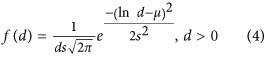The solid curves in Fig. 4b and 4c represent the fitted log-normal distribution function and the extracted values of μ and s are labeled. For the size distribution in the statistical analysis, we employ the median diameter D0.5 in the cumulative distribution function F(d) because it can describe a representative value in the asymmetric distribution. F(d) is described as9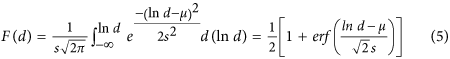where erf (x) is the error function that is written by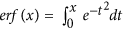. Figure 5 represents the cumulative distribution curves of droplets at 833, 953 and 1073 K and of wires at 773, 953 and 1073 K using the values of μ and s obtained in Fig. 4. D0.5 is defined as the line of F(D0.5) = 0.5, which is shown as the dashed line in Fig. 5 and consequently, D0.5 = eμ. Table 1 shows the summarized results of D0.5 obtained at each temperature. For the droplet, D0.5 = 6.52 μm at 833 K gradually decreases to D0.5 = 3.03 μm at 1073 K. For the wire, D0.5 = 1.53 μm at 773 K suddenly decreases to D0.5 = 0.40 μm at 833 K and the values of D0.5 are almost saturated to D0.5 = 0.39 μm at 1073 K. The thicker wires at 773 K imply that the high viscosity suppresses not only capillary breakup but also ligament elongation.

## Discussion

The results obtained by the statistical analyses are important because they can provide median diameter as evaluation criteria. To understand our experimental data, we have calculated the Ohnesorge number that depends on the temperature as a function of d. For the calculations, the Vogel-Fulcher-Tammann (VFT) relation is applied to the viscosity, η(T) = η0 exp{D*T0/(T - T0)}, where η0 is the viscosity at infinite temperature, D* is a measurement of the kinetic fragility and T0 is the VFT temperature16. From equation (3),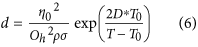Assuming σ = 1 N/m, we use the values of η0 = 4 × 10−5 Pa s, D* = 12 and T0 = 365 K from refs. 17 and 18. We also take account of the temperature dependent ρ in the Pd-MG as ρ = {1.304 × 10−8 T + 1.10 × 10−4}−1 from ref. 21. Figure 6 shows the Ohnesorge curves at Oh = 0.33, 1, 2 and 16, indicating the tendency where the diameter increases with decreasing temperature. The median diameters in Table 1 and the droplet and wire ratios in Fig. 4a are superimposed in Fig. 6. The ratio of droplets significantly decreases at Oh < 1 and it is only 5% (833 K) at Oh = 2. In contrast, the ratio of wires greatly increases at Oh > 1 where it increases from 12% at 1013 K to 44% at 953 K. Furthermore, the sudden increase of diameter to 1.53 μm at 773 K corresponds to Oh = 16. In this stage, the high viscosity suppresses ligament elongation and thus thicker wires dominantly appear.

The experimental results demonstrate that the diameters obey the log-normal distribution that brings us perspectives for theoretical understanding of the atomization process. The log-normal distribution of diameters is originally derived from Kolmogorov’s concept of viewing breakup as a discrete random process22,23. According to the concept, the median is obtained from the products that were produced at the final stage in breakup-cascade processes. To explain the experimental results, it would be necessary to provide a novel phenomenological model beyond the Kolmogorov’s concept. In our experimental situation, the viscosity effect is extremely strong, as seen at Oh > 2. Furthermore, the droplets are rapidly solidified during the deformation process. In contrast, the wire deformation is influenced by both viscosity and solidification. Smoluchowski has also expressed the break-up processes of viscoelastic liquid with a gamma distribution24,25. The difference between the gamma distribution and the log-normal distribution can be identified by the tail in distribution shape. In details, the gamma distribution is derived by taking into account the coalescence of small droplets. However, the coalescences should be a rare event in our experiment because they are rapidly solidified. Thus, the argument on the basis of the D0.5 fitted by the log-normal distribution would be plausible.

To account for the ratio of droplets and wires theoretically, we require further analysis based not only on the diameter distributions but also on wire lengths. Some non-trivial phenomena are still expected to mediate both fragmentation and elongation. For example, the onset of elongation at a droplet occurs when the externally applied force, f  (ex), which should be from the gas jets, exceeds the capillary force, f  (cap). The external force depends on the surface area of a melting droplet. Namely, it is proportional to the square of the characteristic length of a droplet, f  (ex) = α1pd2, where p is the external pressure which is assumed as a given constant and α1 is just a numerical constant. While, the capillary force against the elongation is proportional to the characteristic length itself, f  (cap) = α2σd, where α2 is the numerical constant. Hence, there is a critical diameter dc beyond which a droplet can be elongated. The critical diameter is proportional to the capillary coefficient and inversely proportional to the external pressure, dc = (α21)σ/p. In addition, a droplet is also subjected to the viscous force while elongated. If there is some timescale while which the droplet is subjected to the external force, the final length of the wire (including the droplet) is determined by the viscous force.

In summary, we have studied the formation rule of Pd-MG droplets and wires. The size evaluation of gas atomized products has succeeded with the median diameters which are derived by fitting of the log-normal distribution function and the half value in the cumulative distribution function. We show that the Ohnesorge curves describe the temperature dependence on the diameter which increases with decreasing temperature and the appearance of droplets and wires can be expressed by the Ohnesorge number. There is an implication from this study. To obtain fine nanowires, the condition should be Oh > 2, but the much higher Ohnesorge number leads to a thicker wire. Further, the jet speed that is related to the gas jet pressure during atomization must be investigated26.

## Methods

### Materials Fabrication

We used a custom-built gas atomization system constructed by Nisshin Giken Co. and the experiments were carried out for 5 gram at maximum for alloys or metals. In this experiment, about 3 g of Pd-MG alloy in a quartz crucible was heated by an induction heating system in Ar atmosphere. The end of the quartz crucible had a nozzle with the diameter of 0.1 mm. Tin was monitored by an optical pyrometer (CHINO, IR-CAI3CS) with the emissivity of 0.50, which was calibrated by the melting point of Al in the gas atomization system, as illustrated in Fig. 1. The sample was once heated up to Tin = 1073 K well above the melting point (Tm = 803 K)15. The melt stream was extruded through the nozzle with the injection Ar pressure of 0.05 MPa and was atomized with the Ar gas jet pressure of 10 MPa. After the atomization, all products were accumulated in the bottom of the chamber and the cyclone collector. The total lost of the products was within 5 weight %.

### Materials characterization

Structural and compositional analyses were performed with scanning electron microscopy (JEOL JSM-7800F), energy dispersive X-ray spectroscopy (Oxford Instruments X-Max 50) and X-ray diffraction (Rigaku SmartLab 9MTP). The thermal properties were measured by differential scanning calorimeter (Rigaku DSC8230), which was carried out with the heating rate of 20 K/min. For the statistical analysis, we used OriginPro 9.1 (OriginLab). For the analysis of droplet and wire diameters, imGauge (Imsoft Co., Ltd.) was used.

How to cite this article: Yaginuma, S. et al. Log-normal diameter distribution of Pd-based metallic glass droplet and wire. Sci. Rep. 5, 10711; doi: 10.1038/srep10711 (2015).

## References

1. Kumar, G., Desai, A. & Schroers, J. Bulk metallic glass: The smaller the better. Adv. Mater. 23, 461–476 (2011).

2. Kumar, G., Tang, H. X. & Schroers, J. Nanomoulding with amorphous metals. Nature 457, 868 (2009).

3. Carmo, M. et al. Bulk metallic glass nanowire architecture for electrochemical applications. ACS Nano 22, 2979–2983 (2011).

4. Nakayama, K. S. et al. Metallic glass nanowire. Nano Lett. 8, 516 (2008).

5. Nakayama, K. S. et al. Controlled formation and mechanical characterization of metallic glassy nanowires. Adv. Mater. 22, 872–875 (2010).

6. Nakayama, K. S., Yokoyama, Y., Wada, T., Chen, N. & Inoue, A. Formation of metallic glass nanowires by gas atomization. Nano Lett. 12, 2404–2407 (2012).

7. Nakayama, K. S. et al. Ferromagnetic resonance in soft-magnetic metallic glass nanowire and microwire. Appl. Phys. Lett. 105, 202403 (2014).

8. German, R. M. in Powder metallurgy science (Metal Powder Industries Federation, 1994).

9. Bayvel, L. & Orzechowski, Z. in Liquid atomization (Taylor and Francis, 1993).

10. Nukiyama, S. & Tanasawa, Y. Experiments on the atomization of liquids in an air stream. Report III: On the droplet-size distribution in an atomized jet. Trans. Jpn. Soc. Mech. Eng. 5, 62–67 (1939).

11. Shimozuru, D. Physical parameters governing the formation of Pele’s hair and tears. Bull. Volcanol. 56 217–219 (1994).

12. Eggers, J. & Villermaux, E. Physics of liquid jets. Rep. Prog. Phys. 71, 036601 (2008).

13. Villermaux, E. The formation of filamentary structures from molten silicates: Pele’s hair, angel hair and blown clinker. C. R. Mecanique 340, 555–564 (2012).

14. McKinley, G. H. & Renardy, M. Wolfgang von Ohnesorge. Phys. Fluids 23, 127101 (2011).

15. Nishiyama, N. & Inoue, A. Glass-forming ability of Pd42.5Cu30Ni7.5P20 alloy with a low critical cooling rate of 0.067 K/s. Appl. Phys. Lett. 80, 568–570 (2002).

16. Angell, C. A. Formation of glasses from liquids and biopolymers. Science 267, 1924–1935 (1995).

17. Schroers, J. Processing of bulk metallic glass. Adv. Mater. 22, 1566–1597 (2010).

18. Schroers, J. On the formability of bulk metallic glass in its supercooled liquid state. Acta Mater. 56, 471–478 (2008).

19. Haruyama, O. et al. Thermodynamic approach to glass-forming ability of water-quenched Pd-P-based and Pt60Ni15P25 bulk metallic glasses. Phys. Rev. B 83, 064201 (2011).

20. Babinsky, E. & Sojka, P. E. Modeling drop size distributions. Prog. Energ Combust. 28, 303–329 (2002).

21. Nishi, T. et al. Electrical resistivity measurements of Pd40Cu30Ni10P20 alloy in supercooled liquid and liquid state. Mater. Trans., JIM 45, 2584–2586 (2004).

22. Kolmogorov, A. N. On the log normal distribution of the fragment sizes under breakage. Dokl. Akad. Nauk SSSR 31, 99–101 (1941).

23. Rimbert, N. & é ero-Guillaume, O. Log-stable laws as asymptotic solutions to a fragmentation equation: Application to the distribution of droplets in a high Weber-number spray. Phys. Rev. E 69, 056316 (2004).

24. Villermaux, E., Marmottant, Ph. & Duplat, J. Ligament-mediated spray formation. Phys. Rev. Lett. 92, 074501 (2004).

25. Villermaux, E. Unifying ideas on mixing and atomization. New J. Phys. 6, 125 (2004).

26. Zheng, B. & Lavernia, E. J. in Handbook of atomization and sprays-theory and applications (ed. Ashgriz, N. ) 837–848 (Springer, 2011).

## Acknowledgements

We thank O. Haruyama for providing Pd-based metallic glass, H. Kato, Y. Yamazaki and T. Nakamura for stimulating discussions and R. Kumashiro and K. Saito for technical assistance. This work has been partly supported by JSPS Grant-in-Aid for Scientific Research (B) # 25286019, Young Scientists (B) # 26870058, JST A-STEP #H25 S II-463 and WPI Fusion Research Program.

## Author information

K.S.N conceived the idea of the research. S.Y., C.N. and K.S.N. wrote the manuscript. S.Y. and N.K. carried out the fabrication and the characterization of droplets and wires. Y.Y. and K.S.N designed and constructed the instrumentation. C.N. led the statistical analysis. All the authors read the manuscript, provided comments and approved the content.

## Ethics declarations

### Competing interests

The authors declare no competing financial interests.